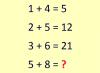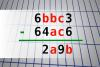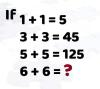BRAIN TEASERSBrain Teasers User Profile

# leslie looman

rank
524
points
3
See full ranking list
short ranking list
 522 mark cevaal 3 523 Suresh kumar 3 524 leslie looman 3 525 Dolores Smoljanovic 3 526 Eduardo Moreira 3
 Calculate 5+8 If 1+4=5, 2+5=12 and 3+6=21 then 5+8=?Find number abc If 6bbc3 - 64ac6 = 2a9b find number abc. Multiple solutions may exist.Mathematical Puzzle: IF 1+1=... Mathematical Puzzle: IF 1+1=5, 3+3=45 and 5+5=125 THEN 6+6=?